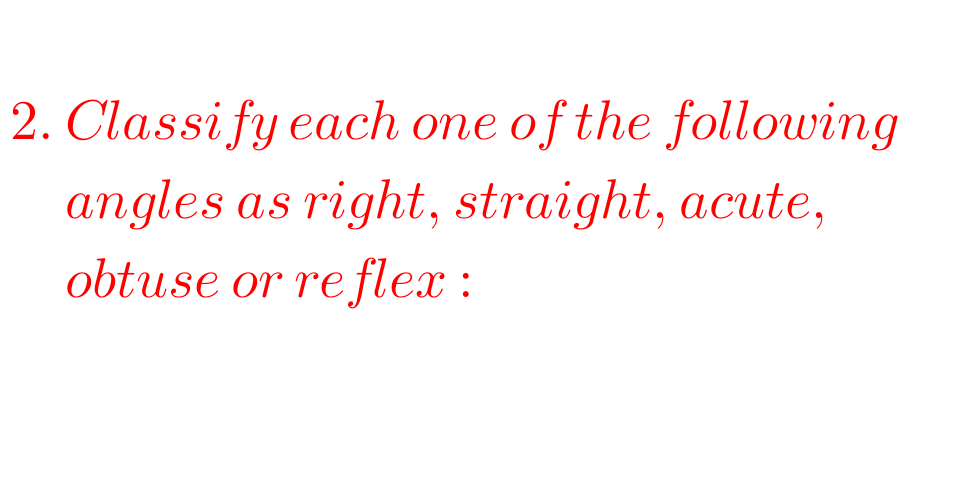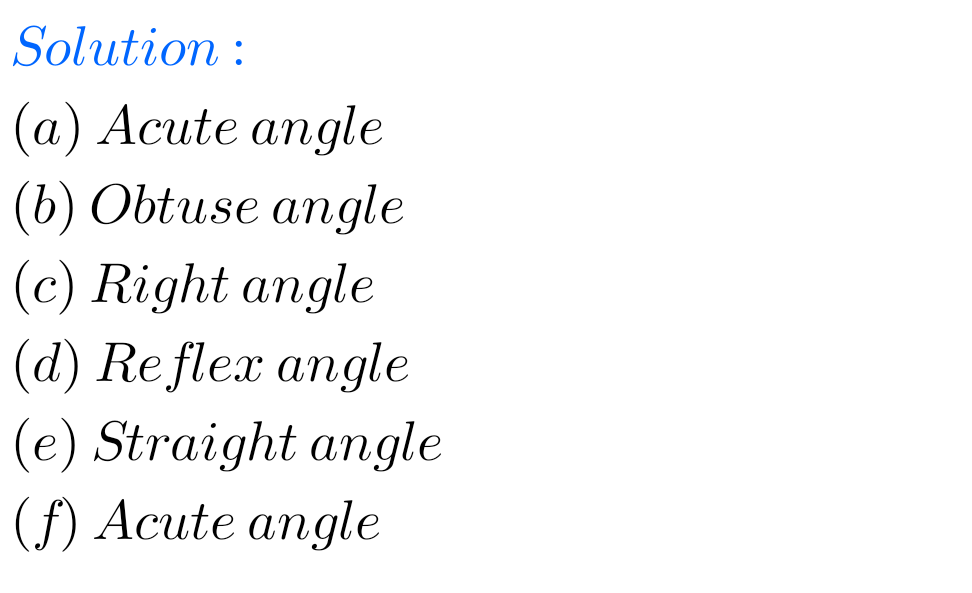# Maths solutions for Understanding Elementary Shapes Chapter 5 exercise 5.3 Ncert class 6

Ncert Maths Class 6 chapter 5 Understanding Elementary Shapes Exercise 5.3 solutions are given.

Study the textbook lesson Understanding Elementary Shapes very well.

Observe the example problems and solutions given in the textbook.

Observe the solutons and try them in your own method.

You can see

Ncert maths solutions for class 6
Understanding elementary shapes

Exercise 5.1

Exercise 5.2

Exercise 5.3

Exercise 5.4

Exercise 5.5

Exercise 5.6

Exercise 5.7

Exercise 5.8

Exercise 5.9

M

M

# Exercise 5.3 chapter 5 class 6 Ncert Maths

Chapter 5

Understanding Elementary Shapes

Exercise 5.3## Maths solutions Ncert Class 6 maths Exercise 5.3 Understanding Elementary ShapesF

## Chapter 5 Understanding  Elementary Shapes Exercise 5.3 solutions Ncert class 6 MathsNote : Observe the solutions and try them in your own method.